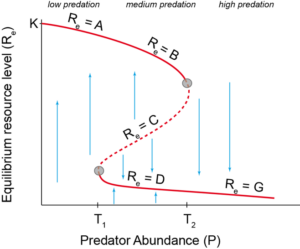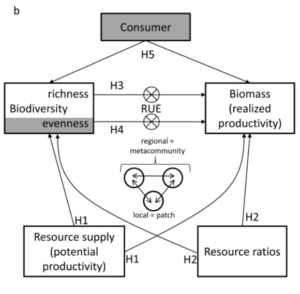## Theoretical Ecology

The course is designed to present an overview of some of the more basic concepts in theoretical ecology, and an examination of some of the more subtle ideas.  For example, we will begin with an examination of the logistic and exponential growth equations.  However, we will also examine what the introduction of time lags does to the predictable behavior of the continuous time model, and see how easily chaos raises its ugly head in the discrete-time formulation of logistic growth.  The course will start with theory at the population level, then move on to such topics as island biogeography, metacommunities, and the interaction of diversity and stability in ecosystems.  Because all of the theory and much of the literature emphasizes a mathematical approach, we too will deal with mathematics, but stress a graphical approach in order to get an intuitive feel for the dynamics of the equations.  In order to show how simple it is to work with mathematically-explicit models, the practical part of the course will develop several of the models mentioned in the lecture using the computer program R.

#### Course content:

Exponential and logistic growth rates in continuous and discrete time, projection matrices and their application, life history strategies, competition and predation models, island and insular biogeography, metapopulations and metacommunities, the neutral theory of biodiversity, diversity and stability in ecosystemsNach May (1977)                                Hillebrand & Lehmpfuhl (2011)                  Holyoak et al. (2005)

“All models are wrong.  Some are useful.”

George Box